We use cookies to enhance your experience on our website. By continuing to use our website, you are agreeing to our use of cookies. You can change your cookie settings at any time. Find out more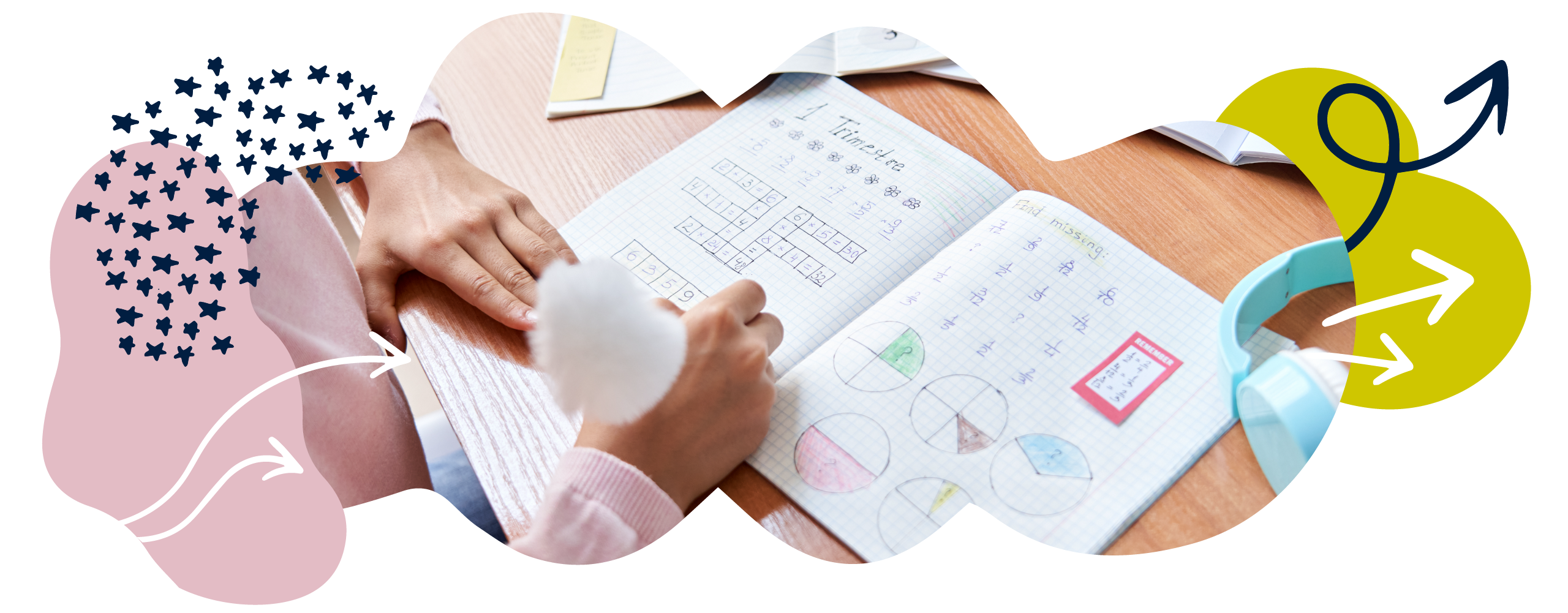# Fractions in Year 3 (age 7–8)

In Year 3, your child will learn about tenths, unit fractions and non-unit fractions, and will begin to understand equivalent fractions (fractions that have the same value such as  = ).

The key words for this section are unit fractiondenominatornumerator, and number line.

## What your child will learn

Take a look at the National Curriculum expectations for fractions in Year 3 (age 7–8):

#### Understand and count in tenths

In Year 3, your child will learn to count in tenths. They will understand that finding a tenth is the same as dividing by ten (by dividing objects, sets of objects, and numbers into ten equal parts).

This will help them to keep seeing the connection between fractions and division. It is possible that your child will start to be introduced to decimals at this stage, too.

#### Find fractions of a set of objects

Your child will learn to find and write fractions of a given number of objects. For example:

Find  of 15.

First, your child can find  of 15 by dividing the total by the denominator:

15 ÷ 5 = 3.

Then, they can multiply this result by the numerator to find the correct fraction:

If  of 15 is 3, then  of 15 is 3 × 2 = 6.

of 15 is therefore 6.

Your child will be able to do this with unit and non-unit fractions. They will learn that:

• A unit fraction is any fraction with a numerator of 1 (for example, )
• A non-unit fraction is any fraction with a numerator larger than 1 (for example, ).

#### Use fractions as numbers

Your child will use fractions as number in their own right. For example, they will be able to find  on a number line and to show that it is a number halfway between 0 and 1.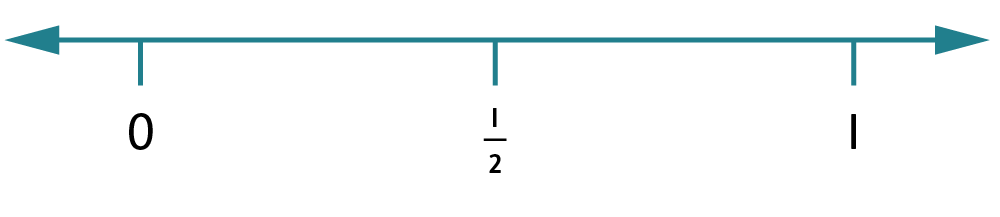Your child will be able to do this with unit and non-unit fractions:

• A unit fraction is any fraction with a numerator of 1 (for example,).
• A non-unit fraction is any fraction with a numerator larger than 1 (for example, ).

At this stage, they will work with fractions with small denominators. This means fractions like  and  rather than fractions with large denominators, like  or .

#### Show equivalent fractions using diagrams

Your child will be able to recognise equivalent fractions with small denominators from diagrams. For example, from the diagram below, children should be able to see that   is equivalent to .

They’ll show their understanding of equivalent fractions by drawing diagrams like this: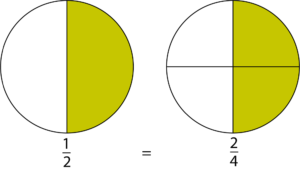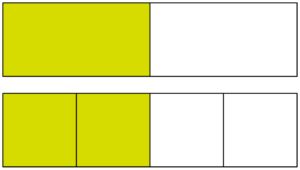Your child will also learn to use objects and fraction walls to show equivalent fractions.

#### Add and subtract fractions with the same denominator

Your child will be encouraged to use drawings and diagrams, as well as symbols, to add and subtract fractions with the same denominator (for example,  + ).At this age, they will only be expected to add fractions that have a total sum of 1 or less. For example, they would not be expected to add  +  because that equals 1 , which is greater than 1.

#### Compare and order fractions

Your child will learn to compare unit fractions (fractions with a numerator of 1) and fractions with the same denominator. They will be able to say which fraction is bigger or smaller and why.

They will be able to put a list of these kinds of fractions in order (for example, biggest to smallest).

#### Solve problems that involve all of the above

Finally, your child will learn to put all this learning into practice by solving problems involving fractions.

They will be able to relate fractions to division, count in fractional steps (for example, counting up and down in tenths), compare fractions, understand unit and non-unit fractions, understand equivalent fractions, find fractions of amounts, and add and subtract fractions with the same denominator.

## How to help at home

There are lots of everyday ways you can help your child to understand fractions. Here are just a few ideas.

### 1. Make fraction snowflakes

Paper folding can be a great way to explore fractions.

Decorate a piece of paper or card and then ask your child to fold the paper into different equal parts. For example, they could fold the paper into fifths by creating five equal sections. Ask them to show you a fifth, two-fifths, three-fifths, four-fifths, and five-fifths, which they can see is equivalent to a whole.

Challenge your child by giving the paper a value and asking them to work out what the fractions would be worth. What would one fifth represent if the whole paper had a value of 10? As they fold, they may like to cut patterns into the paper to make a ‘snowflake’ and watch the pattern develop as they fold further.

### 2. Have fun with food

Cake, pizza, or any foods with a regular shape can help children understand what fractions are and how they work. Ask your child questions like, ‘If I cut our cake into 8 pieces, what fraction will each piece be?’

Foods that people eat lots of can help your child understand how to find fractions of amounts. For example:

‘We have 12 fish fingers in the packet. There are 4 of us. What fraction of the fish fingers can we each have? How many fish fingers would that be?’

### Activity: Cupcake fractions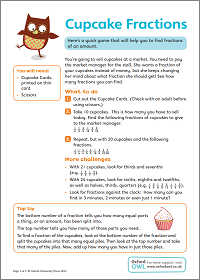Practise finding fractions of amounts with this fun game.

### 3. Compare fractions together

In Year 3, your child will compare unit fractions and fractions with the same denominator by saying which fraction is bigger or smaller – and explaining how they know.

You could show your child fractions like  and  and ask them to explain which fraction is bigger. Many children may incorrectly believe a fifth is bigger than a third because they see a five and know that five is bigger than three. Your child should realise that, with unit fractions, the larger the denominator, the smaller the fraction.

Explain that the whole is divided into 5 equal parts when we talk about a fifth, but 3 equal parts when we talk about a third. If the whole was a cake, you would have less if you had  than if you had  (demonstrate this with a real cake!). By drawing diagrams like the one below, your child will be able to see that  is larger than .### 4. Fraction countdown

Your child will be expected to put unit fractions in order. When ordering unit fractions, help your child by asking them to think of the whole as an object, such as a cake or plate of sandwiches. This should help them to see that  is smaller than . For example:

‘Would you prefer it if I cut this cake into ten equal pieces and gave you a piece, or if I cut the cut into two equal pieces and gave you a piece?’

Print out the Fraction Match activity sheet and cut out each fraction card. Take a selection of cards and see if your child can put them all in order, either from largest to smallest or smallest to largest. Are there any equivalent fractions? See if your child can match them up.

### Activity: Fraction Match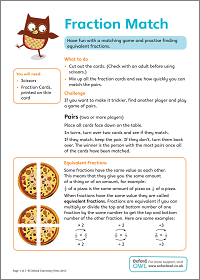Have fun with a matching game and practise finding equivalent fractions.

### 5. Make a fraction wall

Equivalent fractions are different fractions that have the same value. In Year 3, your child will learn about equivalent fractions with small denominators using drawings and diagrams.

Fraction walls are a helpful way to explore equivalent fractions. They show fractions visually, which helps children to see that some fractions that are written differently nonetheless have the same value (for example,  has the same value as , and so on).

Why not try making a fraction wall with your child? You could investigate using the computer to make your fraction wall by creating a table and splitting each row into the equal number of parts you need. For example, to represent fifths, you would have to split the whole row into five equal parts.

### Fraction wall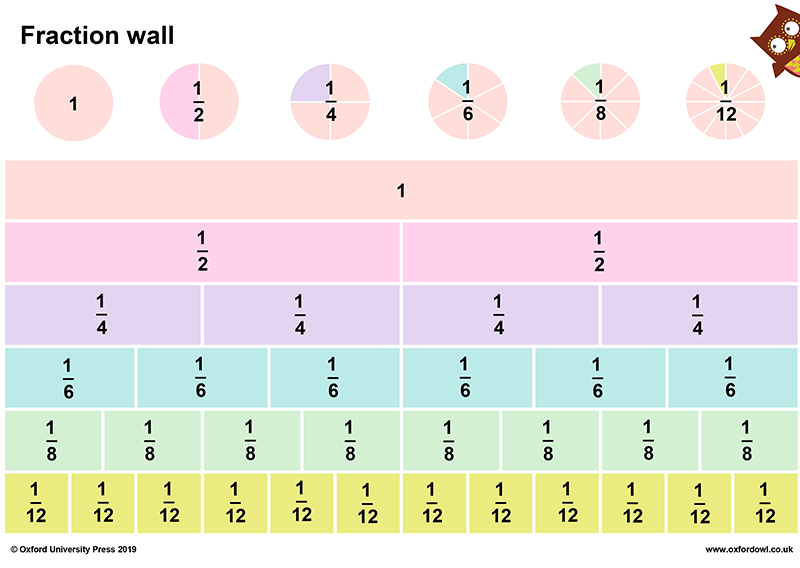Stick our fraction wall on the fridge!

### 6. Adding and subtracting fractions

Try adding and subtracting fractions with the same denominator with your child. Try to do this within a whole, i.e. make sure that the sum uses numbers less than one, including the answer.

+  =

This could be calculated using a diagram like this: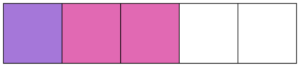Your child will first split the whole into five equal parts. Your child can then shade one box to represent  and two boxes to represent . They will then be able to see that the total is .